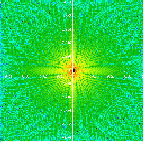### Developed Surface and Length

This criteria is determined by calculating the real area of the surface or the real length of the profile. It is then expressed in percentage area at the base by :

Ld=100·Lreall / NxPx
Sd=100·Sreal / (NxPxNyPy)

### Calculating Peaks or Hollows (valleys) – Retention Volume

A specific function has been developed to count peaks or valleys. From a given altitude (Zref), the volumes (Vp, Vv) and the sections which are associated with them (Sp, Sv) can be calculated for each peak or valley.A more global approach has also been developed to determine the evolution of the retention volume (Vr) in function of the altitude by :

This type of approach is particularly interesting when describing either surfaces involved in lubrified environments, or contact areas involving more or less rubbery materials which are in contact with more or less rigid materials

### R and W Criteria (MOTIF)

The above criteria “R” and “W” are based on a geometrical empirical approach, developed by and for the French automobile industry and meant to study rough profiles.

The standardized reference number is A32 6100.

This approach firstly determines the intuitive motifs of roughness. A motif can show a segment of a profile with two major peaks which serve to calculate the AR steps and the R amplitude of roughness. An “envelope” profile joining these peaks is thus obtained. This envelope, in turn, allows to determine the waviness motifs and thus to calculate the W steps and the waviness amplitudes.### FREQUENTIAL PARAMETERS

By using the Fast Fourier Transform, directly applied to the discretized signal z(x,y) or z(x), one can determine the Power Spectral Density PSD (fx,fy), and the auto-correlation function C(bx,by) from which the frequential parameters can be studied.DSP(fx,fy)= |TF[z(x, y)] | 2C(bx , by) = TF[DSP]